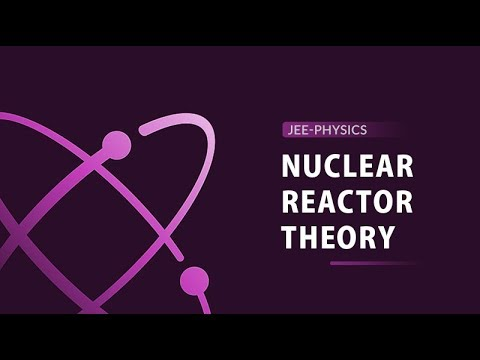# Artificial Transmutation

Transmutation or nuclear transmutation is a process that entails a change in the nucleus of an atom This transmutation process can be either natural or artificial. The natural transmutation process occurs in unstable radioactive elements. On the other hand, artificial transmutation occurs when atoms of one element are struck with fundamental particles. In this article, we will be familiarizing ourselves with artificial transmutation.

## What is Artificial transmutation?

Artificial transmutation is the conversion of an element into another element by bombarding it with a fundamental particle. Artificial transmutation was first carried out on Nitrogen whose nucleus was bombarded with an α particle to produce oxygen. The element that is produced exhibits radioactivity resulting in induced radioactivity.

Following are the particles that are used for bombardment:

• α-particle (2He4)
• Proton (1H1)
• Deuteron (1H3)
• Neutron (0n1)

Since α-particles, protons, and deuterons carry positively charged nuclei, they repel positively charged nucleus and are not suitable projectiles. On the other hand, neutrons possess no charge at all and are considered to be the best projectiles. When a neutron bombards a target element, the resulting product depends upon the speed of the neutrons. Slow neutrons penetrate the nucleus while high-speed neutrons pass through the nucleus. The slow neutrons are known as thermal neutrons and are more effective than the high-speed neutrons in producing nuclear reactions.

## Examples of Artificial Transmutation

Following are some of the examples of artificial transmutation:

• Nitrogen can be transformed into oxygen by bombarding an alpha particle into the nucleus of nitrogen. An atom of hydrogen is produced as part of the transformation.

$$\begin{array}{l}_{7}^{14}\textrm{N}+_{2}^{4}\textrm{He}\rightarrow _{8}^{17}\textrm{O}+_{1}^{1}\textrm{H}\end{array}$$

•

• Aluminum is transformed into phosphorous by combining the nucleus with an alpha particle. A neutron is produced as part of the transformation.

$$\begin{array}{l}_{27}^{13}\textrm{Al}+_{4}^{2}\textrm{He}\rightarrow _{30}^{15}\textrm{P}+_{1}^{0}\textrm{n}\end{array}$$
• From the above artificial transmutation examples it is clear to us that the three “conservation laws” apply to nuclear reactions:

It is important to note that the three “conservation laws” apply to nuclear reactions:

1. The charge is conserved, or, the sum of charges on the left is equal to the sum of charges on the right.
2. The number of nucleons in a nuclear reaction is conserved.
3. The mass-energy relation is conserved.

## Artificial Transmutation Equation

An equation represents the conversion of one element to another. The artificial transmutation is shown with the number of protons. The element which is to be converted is kept on the left side along with the bombarding fundamental particles, while the final element is written on the right side along with any subatomic particles.

For example, Uranium -238 is bombarded with a neutron and is converted to Uranium – 239, which is very unstable, therefore decays to Neptunium emitting beta particle.

$$\begin{array}{l}_{92}^{238}\textrm{U}+_{0}^{1}\textrm{n}\rightarrow _{92}^{239}\textrm{U}\end{array}$$

$$\begin{array}{l}_{92}^{239}\textrm{U}\rightarrow _{93}^{239}\textrm{Np}+_{0}^{-1}\textrm{e}\end{array}$$

 Related Articles:

## Artificial Transmutation Chemistry

The radioactive isotopes resulting from the transmutation is known as tracer elements. Tracer elements are used to study the environmental effects of some pesticides and are also used for medical purposes. The excess of phosphorus in plants is traced by calcium phosphate-containing radioactive isotope of phosphorus.

The artificial transmutation of transuranic elements has helped to manage radioactive waste. It is done by reducing the proportion of those nuclei which has a significant half-life period. These nuclei are made to undergo fission reactions by bombarding them with fast-moving neutrons. In this way, the half-lives of the isotopes of plutonium and other actinides which are many thousands of years can be reduced due to the fission reaction. The resulting fission products are shorter-lived with fewer half-lives (of almost less than 30 years).

Therefore, the hazardous effects of nuclear waste can be minimized by replacing the longer-lived actinides with shorter-lived ones. Although the resulting products are radioactive, their radioactivity will decay in a short period.

## Frequently Asked Questions – FAQs

### What is meant by Artificial Transmutation?

Artificial transmutation is the conversion of an element into another element by bombarding it with a fundamental particle.

### What is an example of Artificial Transmutation?

Aluminium is transformed into phosphorous by combining the nucleus with an alpha particle. A neutron is produced as part of the transformation.

### What is Artificial Transmutation used for?

The artificial transmutation of transuranic elements has helped to manage radioactive waste. It is done by reducing the proportion of those nuclei which has a significant half-life period.

### What is required for Artificial Transmutation to occur?

Artificial transmutation occurs when atoms of an element are struck with fundamental particles such as an alpha particle.

### What happens in an artificial transmutation of an element?

In artificial transmutation, a non-radioactive element is converted into a radioactive element by bombarding it with a fundamental particle through artificial means.

Learn about the theory of nuclear reactors by watching this engaging video!